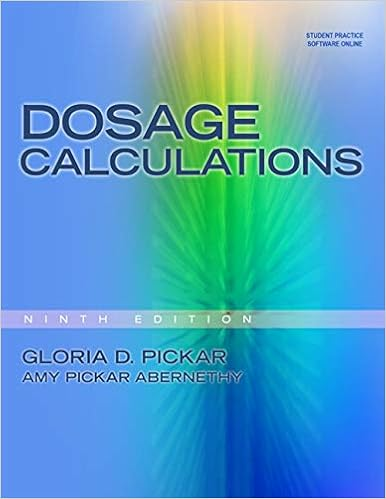# The converse is also true if points d e and f are

• 172
• 92% (12) 11 out of 12 people found this document helpful

This preview shows page 33 - 38 out of 172 pages.

##### We have textbook solutions for you!
The document you are viewing contains questions related to this textbook.The document you are viewing contains questions related to this textbook.
Chapter 2 / Exercise 5
Dosage Calculations
PickarExpert Verified
The converse is also true: If points D , E and F are chosen on BC , AC and AB respectively so that BF AF CD BD AE CE = 1, then AD , BE and CF are concurrent. See Lecture “ Geometry Menelaus and Ceva ” for details. Example 16 : 2 2 b a , 2 2 4 b a , 2 2 4 b a are three sides of a triangle, where a , b , and c are positive numbers. Find the area of the triangle. Solution: We observe that the lengths of the three sides are 2 2 b a , 2 2 ) 2 ( b a , and 2 2 ) 2 ( b a . We can construct a rectangle ABCD such that EF = 2 2 b a , EC = 2 2 ) 2 ( b a , and CF = 2 2 ) 2 ( b a . Therefore, the area of the triangle will be: CEF S = ABCD S AEF S BCE S CDF S = 4 ab 2 1 ab ab ab = 2 3 ab .
##### We have textbook solutions for you!
The document you are viewing contains questions related to this textbook.The document you are viewing contains questions related to this textbook.
Chapter 2 / Exercise 5
Dosage Calculations
PickarExpert Verified
50 AMC Lectures Chapter 10 Area And Area Method 206 Example 17 : Find the area of triangle ABC if three medians are known. Solution: We know that AP = 3 2 AD . So APF = 2 1 ABP = 6 1 ABC . Take M , the midpoint of AP and connect FM : a m MP 3 1 , c m FP 3 1 , b m FM 3 1 MPF S = 2 1 APF S = 12 1 ABC S By Heron’s Formula, we have 12 1 ABC S = MPF S = ) )( )( ( 9 1 c b a m m m m m m m where m = ) ( 2 1 c b a m m m . Therefore, ABC S = ) )( )( ( 3 4 c b a m m m m m m m . Example 18 : In triangle ABC, AD, BE, and CF meet at O. Let the areas of six small triangles be p, q, r, m, n, and k, respectively, as shown in the figure. Show that pqr = mnk (1) k n m r q p 1 1 1 1 1 1 (2) Solution: We begin by proving (1) first: From Example 15, we proved Ceva’s Theorem, which stated: 1 EA CE DC BD FB AF (3) By Theorem 6, we have k p FB AF (4)
50 AMC Lectures Chapter 10 Area And Area Method 207 m q DC BD (5) n r EA CE (6) Substituting (4), (5), and (6) into (3) yields 1 n r m q k p pqr = mnk Now we will prove (2): By Theorem (5), we have: r n k p CD BD m q qn + qr = mp + mk (7) Similarly we get rp + rk = nq + nm (8) pq + pm = kn + kr (9) (7) + (8) + (9): qr + rp + pq = kn + mk + nm (10) Dividing left hand side by pqr and right hand side by mnk : k n m r q p 1 1 1 1 1 1 .
50 AMC Lectures Chapter 10 Area And Area Method 208 PROBLEMS Problem 1 : D and C trisect the arc of the half circle as shown in the figure. Find the shaded area if the area of the half circle is 9 . Problem 2 : As shown in the figure, right triangle ABC with BC = 20 cm. BDC is a half circle with the diameter BC . The difference between two shaded areas I and II is 23. Find AC in terms of . Problem 3: The larger one of the two squares in the figure below has the side length of 10 cm . Find the shaded area. Problem 4 : As shown in the figure, in ABC, D is any point on AB. DE//BC and meets BC at F. The area of ADE is S 1. The area of DBF is S 2 . Find the area of parallelogram DFCE.
50 AMC Lectures Chapter 10 Area And Area Method 209 Problem 5 : In trapezoid ABCD, The area of ABC is 253 cm 2 . The area of AOB is 42 cm 2 larger than the area of COD. Find the area of trapezoid ABCD.
•••